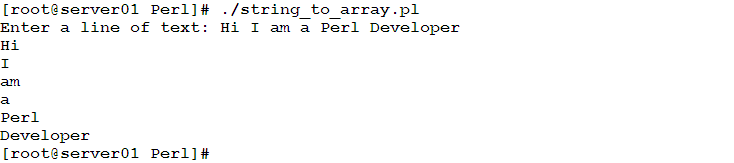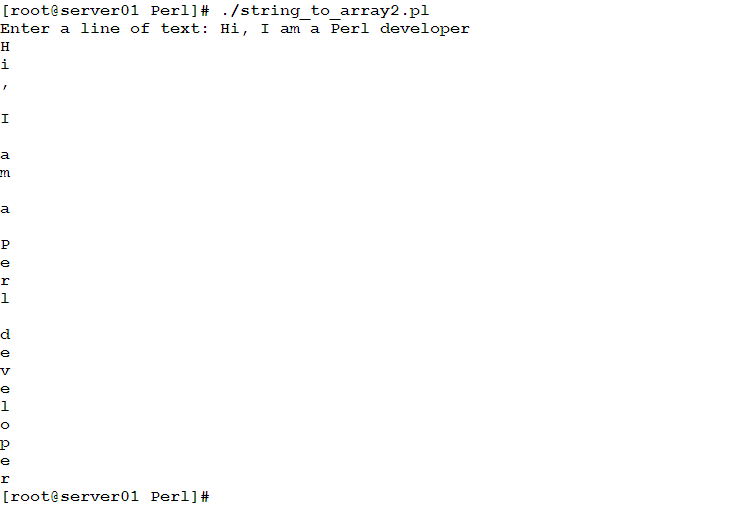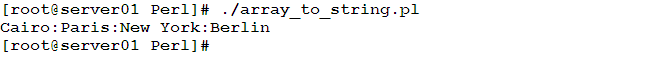Home Perl Programming Learn the relation between Arrays and Strings in Perl Programming

# Learn the relation between Arrays and Strings in Perl Programming

0
4307Welcome to part two of the Arrays topic. In this article, we are going to talk about the relation between Arrays and Strings. In Perl, an array could be easily converted to a string, and vice versa. A short, yet interesting topic, so don’t miss it!!

Converting Strings to Arrays
A string can be converted into an array using the split() function.

Syntax

`@ARRAY = split (/REGEX/, \$STRING);`

Where:
@ARRAY is the array variable that will be assigned the resulting array.
\$STRING is the string to be splitted.
REGEX is the string (pattern) to be matched, and to split the \$STRING accordingly.

Example
In this example, we are going to write a script that prompts the user to enter a line of text, and then splits that line into words. The individual words will be assigned to an array. Finally, the array elements will be printed, one per line.

```#!/usr/bin/perl
print ("Enter a line of text: ");
\$str = <stdin>;
chomp \$str;
@array = split (/ /, \$str);
foreach (@array)
{
print ("\$_\n");
}
```

When executed, the program should behave as follows:The user input is accepted using the <stdin> operator, and then assigned to the \$str variable. The chomp() function removes the trailing newline character from the input string. To extract the individual words from a line of text, the split() function will match the whitespaces (knowing that words in a sentence are separated by whitespaces). The resulting list of words will be assigned to the array variable @array. To loop on the list of elements in an array, the best way is to use the foreach loop (as we know from the previous article).

Extracting Individual Characters in a String
Using the split() function with empty search string “” returns the list of individual characters in a given string.

Example
Re-write the previous example to split the input string into characters (instead of words), and print the characters, one per line.

```#!/usr/bin/perl
print ("Enter a line of text: ");
\$str = <stdin>;
chomp \$str;
@array = split (//, \$str);
foreach \$ch (@array)
{
print ("\$ch\n");
}
```

When executed, it will print the characters as required:Similarly, the split() function can be used to process the /etc/passwd file (in UNIX and Linux systems). In this case, the search pattern will be /:/
I will leave this for the reader as an exercise.

Converting Arrays to Strings
The opposite operation could be done using the join() function.

Syntax

`\$STRING = join (\$CONNECTING_STRING,@ARRAY);`

Where:
@ARRAY: is the input array.
\$CONNECTING_STRING is the string to be used to connect the individual array elements.
\$STRING is the resulting string.

Example
Having the array @cities that contains names for some world’s famous cities, we need to produce a string that contains all the array elements, separated by colon “:”

```#!/usr/bin/perl
@cities = ("Cairo", "Paris", "New York", "Berlin");
\$str = join(":", @cities);
print ("\$str\n");
```

Executing this Perl script gives the following output:Concatenating Arrays
Two or more arrays could be concatenated simply using the following statement:

`@BIGARRAY = (@ARRAY1,@ARRAY2,…);`

Example
Assume you have two arrays:

```@zero2four = (0, 1, 2, 3, 4);
@five2nine = (5, 6, 7, 8, 9);```

To combine the two arrays into a bigger one, use the following statement:

`@numbers = (@zero2four, @five2nine);`

Printing the @numbers array, will produce:

`0 1 2 3 4 5 6 7 8 9`

Summary
In this article, we have looked into the relationship between arrays and strings, and how to convert one type to the other.

• The split() function converts a string to an array.
• The join() function converts an array into a string.

That was part two in the Arrays topic. See you in the next article.